# dsp.FIRRateConverter

Sample rate converter

## Description

The `dsp.FIRRateConverter` System object™ performs sampling rate conversion by a rational factor on a vector or matrix input. The FIR rate convertor cascades an interpolator with a decimator. The rate converter (as shown in the schematic) conceptually consists of an upsampler, followed by a combined anti-imaging and anti-aliasing FIR filter, followed by a downsampler. The coefficients of the anti-imaging and anti-aliasing FIR filter can be specified through the `Numerator` property, or can be automatically designed by the object using the `designMultirateFIR` function. For an example, see Resample Signal Using FIR Rate Converter.

The upsampler increases the sample rate of the signal by a factor L and the downsampler reduces the sample rate of the signal by a factor M. Use upsampling and downsampling factors that are relatively prime or coprime. The resulting discrete-time signal has a sample rate that is L/M times the original sample rate.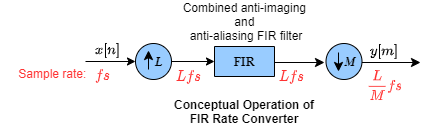Note that the actual object algorithm implements a polyphase structure, an efficient equivalent of the combined system depicted in the diagram. For more details, see Algorithms.

To perform sampling rate conversion:

1. Create the `dsp.FIRRateConverter` object and set its properties.

2. Call the object with arguments, as if it were a function.

## Creation

### Syntax

``firrc = dsp.FIRRateConverter``
``firrc = dsp.FIRRateConverter(L,M)``
``firrc = dsp.FIRRateConverter(L,M,'Auto')``
``firrc = dsp.FIRRateConverter(L,M,num)``
``firrc = dsp.FIRRateConverter(L,M,method)``
``firrc = dsp.FIRRateConverter(___,Name,Value)``
``firrc = dsp.FIRRateConverter(L,M,'legacy')``

### Description

example

````firrc = dsp.FIRRateConverter` returns an FIR sample rate converter object with an interpolation factor of 3 and a decimation factor of 2. The object designs the FIR filter coefficients using the `designMultirateFIR(3,2)` function.```
````firrc = dsp.FIRRateConverter(L,M)` returns an FIR sample rate converter with the integer-valued `InterpolationFactor` property set to `L` and the `DecimationFactor` property set to `M`. The object designs its filter coefficients based on the rate conversion factors that you specify while creating the object, using the `designMultirateFIR(L,M)` function. The designed filter corresponds to a lowpass with normalized cutoff frequency no greater than `min(π/L,π/M)` in radial frequency units.```
````firrc = dsp.FIRRateConverter(L,M,'Auto')` returns an FIR sample rate converter where the `NumeratorSource` property is set to `'Auto'`. In this mode, every time there is an update in the rate conversion factors, the object redesigns the filter using the design method specified in `DesignMethod`.```

example

````firrc = dsp.FIRRateConverter(L,M,num)` returns an FIR sample rate converter with the `InterpolationFactor` property set to `L`, the `DecimationFactor` property set to `M`, and the `Numerator` property set to `num`.```
````firrc = dsp.FIRRateConverter(L,M,method)` returns an FIR sample rate converter with the `InterpolationFactor` property set to `L`, the `DecimationFactor` property set to `M`, and the `DesignMethod` property set to `method`. When you pass the design method as an input, the `NumeratorSource` property is automatically set to `'Auto'`.```
````firrc = dsp.FIRRateConverter(___,Name,Value)` returns an FIR sample rate converter with each specified property set to the specified value. Enclose each property name in quotes. You can use this syntax with any previous input argument combinations.Example: ```firrc = dsp.FIRRateConverter('FullPrecisionOverride','false')``` enables the fixed-point data types to be controlled through the individual fixed-point property settings.```
````firrc = dsp.FIRRateConverter(L,M,'legacy')` returns an FIR sample rate converter where the filter coefficients are designed using `firpm(70,[0 0.28 0.32 1],[1 1 0 0])`. The designed filter has a cutoff frequency of π/3 radians/sample.```

## Properties

expand all

Unless otherwise indicated, properties are nontunable, which means you cannot change their values after calling the object. Objects lock when you call them, and the `release` function unlocks them.

If a property is tunable, you can change its value at any time.

Interpolation factor L, specified as a positive integer.

Data Types: `single` | `double` | `int8` | `int16` | `int32` | `int64` | `uint8` | `uint16` | `uint32` | `uint64`

Decimation factor M, specified as a positive integer.

Data Types: `single` | `double` | `int8` | `int16` | `int32` | `int64` | `uint8` | `uint16` | `uint32` | `uint64`

FIR filter coefficient source, specified as one of the following:

• `'Property'` –– The numerator coefficients are specified through the `Numerator` property.

• `'Auto'` –– The numerator coefficients are designed automatically using the design method specified in `DesignMethod`.

Numerator coefficients of the FIR filter, specified as a row vector in powers of z-1.

The following equation defines the transfer function of the filter:

`$H\left(z\right)=\sum _{n=0}^{N}b\left(n\right){z}^{-n}$`

The vector b = [b(0), b(1), …, b(N)] represents the vector of filter coefficients.

Use a lowpass with normalized cutoff frequency no greater than `min(1/L,1/M)`, where L is the interpolation factor and M is the decimation factor. The gain of the lowpass filter should equal L, the interpolation factor. Choose the length of the filter to be a multiple of the interpolation factor. To design such a filter, use the `designMultirateFIR` function. For an example, see Resample Signal Using FIR Rate Converter. All initial filter states are zero.

#### Dependencies

This property is visible only when you set `NumeratorSource` to `'Property'`.

When `NumeratorSource` is set to `'Auto'`, the numerator coefficients are automatically redesigned using the design method specified in `DesignMethod`. To access the filter coefficients in the automatic design mode, type `objName.``Numerator` in the MATLAB® command prompt.

Data Types: `single` | `double` | `int8` | `int16` | `int32` | `int64` | `uint8` | `uint16` | `uint32` | `uint64`

Design method of the FIR filter coefficients, specified as one of the following:

• `'Kaiser'` –– Kaiser method. Approximate combined anti-aliasing and anti-imaging lowpass filter using the `designMultirateFIR` function.

• `'ZOH'` –– Zero order hold method. Hold the input sequence values.

• `'Linear'` –– Linear interpolation method.

#### Dependencies

This property is visible only when you set the `NumeratorSource` property to `'Auto'`, or if you pass the `'auto'` keyword as an input while creating the object.

### Fixed-Point Properties

Specify whether to use full precision rules. If you set `FullPrecisionOverride` to `true`, which is the default, the object computes all internal arithmetic and output data types using full precision rules. These rules provide the most accurate fixed-point numerics. It also turns off the display of other fixed-point properties because they do not apply individually. These rules guarantee that no quantization occurs within the object. Bits are added, as needed, to ensure that no roundoff or overflow occurs. If you set `FullPrecisionOverride` to `false`, fixed-point data types are controlled through individual fixed-point property settings. For more information, see Full Precision for Fixed-Point System Objects.

Specify the rounding method as one of | `Ceiling` | `Convergent` | `Floor` | `Nearest` | `Round` | `Simplest` | `Zero` |.

#### Dependencies

This property applies only if the object is not in full precision mode.

Specify the overflow action as one of | `Wrap` | `Saturate` |.

#### Dependencies

This property applies only if the object is not in full precision mode.

Specify the filter coefficient fixed-point data type as one of | ```Same word length as input``` | `Custom` |.

Specify the filter coefficient fixed-point type as a `numerictype` (Fixed-Point Designer) object with a `Signedness` of `Auto`.

#### Dependencies

This property applies only when the `CoefficientsDataType` property is `Custom`.

Specify the product fixed-point data type as one of | ```Full precision``` | `Same as input` | `Custom` |.

Specify the product fixed-point type as a scaled `numerictype` (Fixed-Point Designer) object with a `Signedness` of `Auto`.

#### Dependencies

This property applies only when the `ProductDataType` property is `Custom`.

Specify the accumulator fixed-point data type as one of | ```Full precision``` | `Same as product` | ```Same as input``` | `Custom` |.

Specify the accumulator fixed-point type as a scaled `numerictype` (Fixed-Point Designer) object with a `Signedness` of `Auto`.

#### Dependencies

This property applies only when the `AccumulatorDataType` property is `Custom`.

Specify the output fixed-point data type as one of | ```Same as accumulator``` | `Same as product` | ```Same as input``` | `Custom` |.

Specify the output fixed-point type as a scaled `numerictype` (Fixed-Point Designer) object with a `Signedness` of `Auto`.

#### Dependencies

This property applies only when the `OutputDataType` property is `Custom`.

## Usage

### Syntax

``y = firrc(x)``

### Description

example

````y = firrc(x)` resamples the input `x` and returns the resampled signal `y`.```

### Input Arguments

expand all

Data input, specified as a column vector or a matrix. The number of input rows P must be a multiple of the decimation factor M. A P-by-Q matrix input is treated as Q independent channels.

Data Types: `single` | `double` | `int8` | `int16` | `int32` | `int64` | `uint8` | `uint16` | `uint32` | `uint64` | `fi`
Complex Number Support: Yes

### Output Arguments

expand all

Resampled output, returned as a column vector or a matrix. The number of rows in the output signal is given by PL/M, where P is the number of input rows, L is the interpolation factor, and M is the decimation factor.

Data Types: `single` | `double` | `int8` | `int16` | `int32` | `int64` | `uint8` | `uint16` | `uint32` | `uint64` | `fi`
Complex Number Support: Yes

## Object Functions

To use an object function, specify the System object as the first input argument. For example, to release system resources of a System object named `obj`, use this syntax:

`release(obj)`

expand all

 `freqz` Frequency response of discrete-time filter System object `fvtool` Visualize frequency response of DSP filters `info` Information about filter System object `cost` Estimate cost of implementing filter System object `coeffs` Returns the filter System object coefficients in a structure `polyphase` Polyphase decomposition of multirate filter `generatehdl` Generate HDL code for quantized DSP filter (requires Filter Design HDL Coder)
 `step` Run System object algorithm `release` Release resources and allow changes to System object property values and input characteristics `reset` Reset internal states of System object

## Examples

collapse all

Resample a 100 Hz sine wave signal by a factor of 3/2.

Note: If you are using R2016a or an earlier release, replace each call to the object with the equivalent `step` syntax. For example, `obj(x)` becomes `step(obj,x)`.

Create a `dsp.SineWave` object which generates a sinusoidal signal with 54 samples per frame, contains a tone at 100 Hz, and has a sample rate of 1250 Hz.

```sine = dsp.SineWave(1,100,'SampleRate',1250,'SamplesPerFrame',54); % Grab a frame x = sine(); % Calcualte input time vector tx = (0:length(x)-1)/sine.SampleRate; ```

Design Default Filter

Create a `dsp.FIRRateConverter` object. The object uses a combined anti-imaging and anti-aliasing FIR filter. By default, this filter is designed using the `designMultirateFIR` function. The function designs the filter based on the rate conversion factors that you specify, and stores the coefficients in the `Numerator` property. For an interpolation factor of 3 and a decimation factor of 2, the object designs the coefficients using `designMultirateFIR(3,2)`.

`firrc = dsp.FIRRateConverter(3,2);`

Resample by a Factor of 3/2

Resample the signal by a factor of 3/2.

`y = firrc(x);`

Plot the original and resampled signals. In order to plot the two signals on the same plot, obtain the time affine transformation parameters. Use these parameters to compute the output sample times. The ${\mathit{n}}^{\mathrm{th}}$ output sample time equals $\mathrm{scale}×\mathit{n}+\mathrm{delay}$. The input and output values coincide every 3 output samples, and every other input sample, owing to the conversion rate of 3/2.

```scale = firrc.InterpolationFactor/firrc.DecimationFactor; delay = length(firrc.Numerator)/(2*firrc.DecimationFactor); % Calculate output times for vector y in input units ty = (((0:length(y)-1)-delay)/scale)/sine.SampleRate; stem(tx, x,'filled',MarkerSize=4); hold on; stem(ty, y); hold off; xlim([0.0 0.0145]) ylim([-1.5 1.5]) legend('Original input','Resampled');```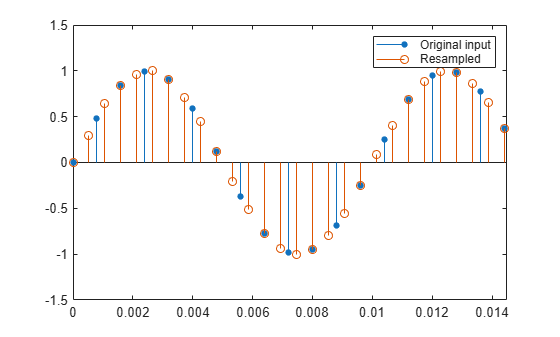Resample by a Factor of 5/3 in Automatic Filter Design Mode

Now change the interpolation factor to 5 and the decimation factor to 3. In order for the filter design to be updated automatically based on the new rate conversion factors, set the `NumeratorSource` property to `'Auto'`. Alternately, you can pass the keyword `'auto'` as an input while creating the object. The object then operates in the automatic filter design mode. Every time there is a change in the rate conversion factors, the object updates the filter design accordingly.

```release(firrc) firrc.NumeratorSource = 'Auto'; firrc.InterpolationFactor = 5; firrc.DecimationFactor = 3```
```firrc = dsp.FIRRateConverter with properties: InterpolationFactor: 5 DecimationFactor: 3 NumeratorSource: 'Auto' DesignMethod: 'Kaiser' Show all properties ```

To access the filter coefficients in the automatic filter design mode, type `firrc.Numerator` in the MATLAB command prompt.

Resample the signal with the updated rate conversion values.

`yAuto = firrc(x);`

Plot the original and resampled signals. Recalculate the time affine transformation parameters since the rate conversion factors have changed. Note the input and output coincide every 3 input samples, and every 5 output samples, owing to the 5/3 conversion factor.

```scale = firrc.InterpolationFactor/firrc.DecimationFactor; delay = length(firrc.Numerator)/(2*firrc.DecimationFactor); % Calculate output times for vector yAuto in input units tyAuto = (((0:length(yAuto)-1)-delay)/scale)/sine.SampleRate; stem(tx, x,'filled',MarkerSize=4); hold on; stem(tyAuto, yAuto,'r'); hold off; xlim([0.0 0.015]) ylim([-1.5 1.5]) legend('Original input','Resampled');```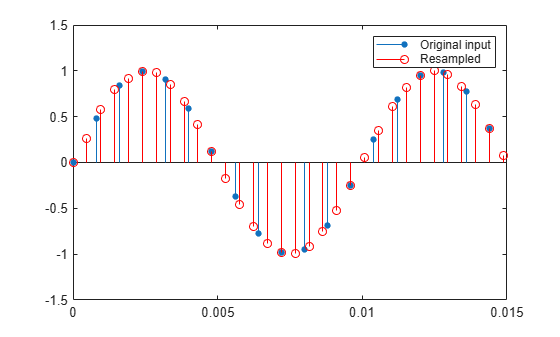Specify Signal Interpolation Model

In the automatic design mode, you can also specify the underlying D/A signal interpolation model through the `DesignMethod` property.

Set `DesignMethod` to `'linear'` and change the interpolation factor to 11.

```release(firrc) firrc.DesignMethod = 'linear'; firrc.InterpolationFactor = 11;```

Resample the signal using the linear interpolation model.

`yLinear = firrc(x);`

Plot the original and resampled signals. The output samples lie on a piecewise-linear curve. Note the input and output coincide every three input samples and every 11 output samples as expected from the ratio 11/3.

```scale = firrc.InterpolationFactor/firrc.DecimationFactor; delay = (length(firrc.Numerator)-1)/(2*firrc.DecimationFactor); % Calculate output times for vector yLinear in input units tyLinear = (((0:length(yLinear)-1)-delay)/scale)/sine.SampleRate; stem(tx, x, 'filled',MarkerSize=4); hold on; stem(tyLinear, yLinear); plot(tyLinear, yLinear,Color=[1 0 0 0.3]); hold off; xlim([0.0 0.009]) ylim([-1.5 1.5]) legend('Original input','Resampled');```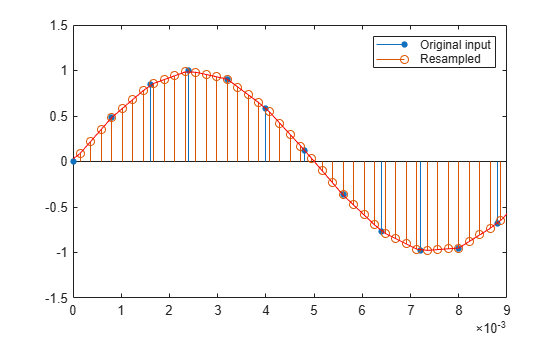Resample an audio signal from 48 kHz to 44 kHz and play the resampled signal using the `audioDeviceWriter` object.

Note: If you are using R2016a or an earlier release, replace each call to the object with the equivalent `step `syntax. For example, `obj(x) `becomes `step(obj,x)`.

Note: The `audioDeviceWriter` System object™ is not supported in MATLAB Online.

Create a `dsp.AudioFileReader` object. The object reads an audio file that has a sample rate of 48 kHz.

```L = 11; M = 12; afr = dsp.AudioFileReader('audio48kHz.wav', ... 'OutputDataType', 'single', ... 'SamplesPerFrame', 4*M);```

Create a `dsp.FIRRateConverter` object with an interpolation factor of L = 11 and a decimation factor of M = 12 (the co-prime representation of the ratio $44\mathit{k}/48\mathit{k}$), a reasonable approximation of the standard 44.1 kHz rate. The object designs the filter using `designMultirateFIR``(11,12)` and stores the coefficients in the `Numerator` property of the object.

`firrc = dsp.FIRRateConverter(L,M)`
```firrc = dsp.FIRRateConverter with properties: InterpolationFactor: 11 DecimationFactor: 12 NumeratorSource: 'Property' Numerator: [0 2.3076e-05 5.4790e-05 9.3620e-05 1.3665e-04 ... ] Show all properties ```

Create an `audioDeviceWriter` object. Specify the sample rate to be 44100 Hz.

`adw = audioDeviceWriter(44100);`

Read the audio file, convert the sample rate of the audio signal, and play the resampled audio.

```while ~isDone(afr) audio1 = afr(); audio2 = firrc(audio1); adw(audio2); end release(afr); release(adw);```

## Algorithms

The FIR rate converter is implemented efficiently using a polyphase structure.

To derive the polyphase structure, start with the transfer function of the FIR filter: This FIR filter is a combined anti-imaging and anti-aliasing filter.

`$H\left(z\right)={b}_{0}+{b}_{1}{z}^{-1}+...+{b}_{N}{z}^{-N}$`

N+1 is the length of the FIR filter.

You can rearrange this equation as follows:

`$H\left(z\right)=\begin{array}{c}\left({b}_{0}+{b}_{L}{z}^{-L}+{b}_{2L}{z}^{-2L}+..+{b}_{N-L+1}{z}^{-\left(N-L+1\right)}\right)+\\ {z}^{-1}\left({b}_{1}+{b}_{L+1}{z}^{-L}+{b}_{2L+1}{z}^{-2L}+..+{b}_{N-L+2}{z}^{-\left(N-L+1\right)}\right)+\\ \begin{array}{c}⋮\\ {z}^{-\left(L-1\right)}\left({b}_{L-1}+{b}_{2L-1}{z}^{-L}+{b}_{3L-1}{z}^{-2L}+..+{b}_{N}{z}^{-\left(N-L+1\right)}\right)\end{array}\end{array}$`

L is the number of polyphase components, and its value equals the interpolation factor that you specify.

You can write this equation as:

`$H\left(z\right)={E}_{0}\left({z}^{L}\right)+{z}^{-1}{E}_{1}\left({z}^{L}\right)+...+{z}^{-\left(L-1\right)}{E}_{L-1}\left({z}^{L}\right)$`

E0(zL), E1(zL), ..., EL-1(zL) are polyphase components of the FIR filter H(z).

Conceptually, the FIR rate converter contains an upsampler, followed by a combined anti-imaging, anti-aliasing FIR filter H(z), which is followed by a downsampler.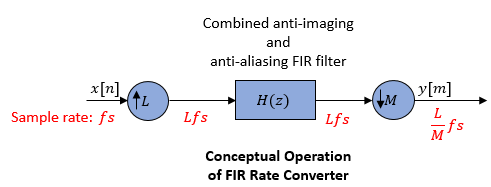Replace H(z) with its polyphase representation.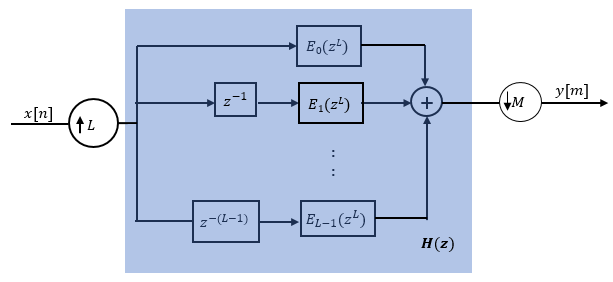Here is the multirate noble identity for interpolation.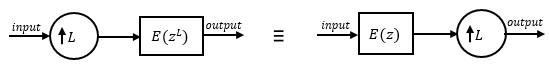Applying the noble identity for interpolation moves the upsampling operation to after the filtering operation. This move enables you to filter the signal at a lower rate.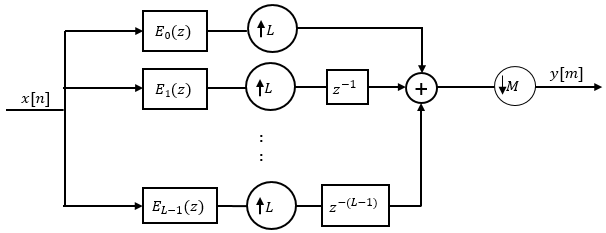You can replace the upsampling operator, delay block, and the adder with a commutator switch. To account for the downsampler that follows, the switch moves in steps of size M. The switch receives the first sample from branch 0 and moves in the counter clockwise direction, each time skipping M−1 branches.

As an example, consider a rate converter with L set to 5 and M set to 3. The polyphase components are E0(z), E1(z), E2(z), E3(z), and E4(z). The switch starts on the first branch 0, skips branches 1 and 2, receives the next sample from branch 3, then skips branches 4 and 0, receives the next sample from branch 2, and so on. The sequence of branches from which the switch receives the data sample is [0, 3, 1, 4, 2, 0, 3, 1, ….].

The rate converter implements the L/M conversion by first applying the interpolation factor L to the incoming data, and using the commutator switch at the end to receive only 1 in M samples, effectively accounting for the dowsampling factor M. Hence, the sample rate at the output of the FIR rate converter is Lfs/M.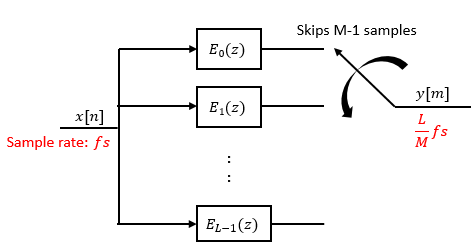## Extended Capabilities

### Fixed-Point ConversionDesign and simulate fixed-point systems using Fixed-Point Designer™.

Introduced in R2012a

## SupportGet trial now